Maths-
General
Easy

Question

# The sides of a triangle are 8cm, 15cm and 17cm. find its perimeter and its area. Also find the length of the altitude drawn on the side with length 17cm.Hint:

## The correct answer is: 7.05 cm

### Let a = 15 cm , b = 8 cm , c = 17 cmPerimeter = Sum of all sides =  a + b + c= 15 + 8 + 17  = 40 cmUsing Heron’s formula,Area of triangle =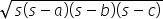where s =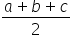s =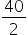= 20Area of triangle =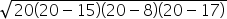=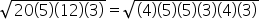= (4)(5)(3) = 60 cm2Also, Area of triangle =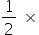b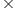h60 =17hh =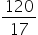⇒ h = 7.05 cm#### With Turito Foundation.#### Get an Expert Advice From Turito.